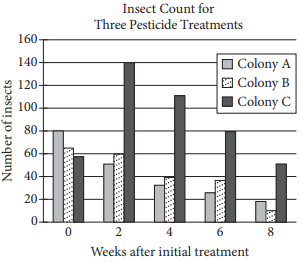mycollegehive
Three colonies of insects were each treated with a differe...
SAT-math-test SAT math0Three colonies of insects were each treated with a different pesticide over an 8-week period to test the effectiveness of the three pesticides. Colonies A, B, and C were treated with Pesticides A, B, and C, respectively. Each pesticide was applied every 2 weeks to one of the three colonies over the 8-week period. The bar graph above shows the insect counts for each of the three colonies 0, 2, 4, 6, and 8 weeks after the initial treatment

Of the following, which is closest to the ratio of the total number of insects in all three colonies in week 8 to the total number of insects at the time of initial treatment?

A) 2 to 5

B) 1 to 4

C) 3 to 5

D) 1 to 2

545 viewsShareFollowUniversity of Lagos Nigeria
04 June 2020University of Benin Nigeria
07 November 20200Number of insect in colony A for week 8 = 18

Number of insect in colony B for week 8 = 10

Number of insect in colony C for week 8 = 52

Therefore, the total number of insect in colonies A, B, and C for week 8 = 18 + 10 + 52 = 80

Number of insect initially in colonies A, B, and C are 80, 62 and 58 respectively

The total of all three colonies are 80 + 62 + 58 = 200

Thus, the ratio of the total number of insect in all three colonies for week 8 to the ratio of total number of insect initially in all three colonies = 80 to 200

= 2 to 5

Ans AShare

### Groups

How to insert math formulas/equations### Related Tags

SAT-math-test

6 followers

476 questionsSAT

3 followers

487 questionsmath

15 followers

1261 questions